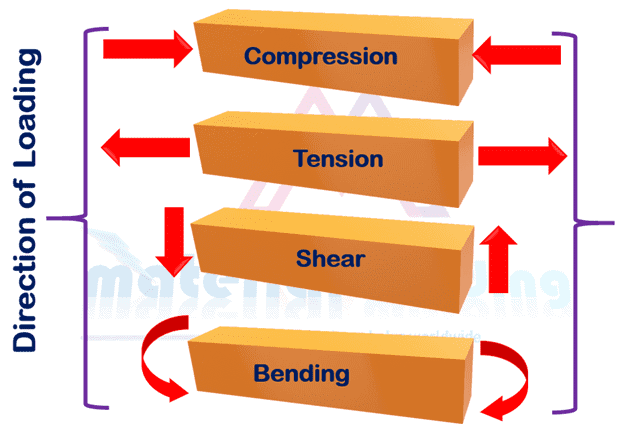# Basics of Welding Design​ & stresses in weld joints

## Basics of Welding Design​

Welding joints are subjected to various types of loading and stresses such as longitudinal, transverse, shear, fatigue and torsional loads as shown in figure 1 and being used for various application e.g. railway, aerospace, oil & gas, structural & heavy fabrication to name a few. The basic for the welding design based on the understanding of these stresses, how they work and affect the component.Figure 1. Various types of stresses in welding structures Various types of stresses in welding structures

The first important consideration for a design is Stress being subjected to the part.

Stress in welding joint is defined as load (or force) divided by the cross-sectional area (CSA) of the component subjected to load. If the force, F, is in newtons (N) and the CSA area in millimeters squared (mm2), then the tensile stress, given the symbol σ, is in newtons per millimeter squared (N/mm2), which is the same as megapascals (MPa).

The stress equation is often written as:

Stress (σ) = Load (F)/ Area (A)

Stresses can act either as a tensile stress (pulling apart) or a compressive stress (squashing together) but is calculated the same way for each, i.e. load over CSA. Tensile stress is often considered dangerous because it requires a tensile stress to spread a crack.

Strain in welding joint: Strain is the change is length caused by the application of a force divided by the original length. Refer figure 3, assuming the original length L, then the change in length is shown as ΔL. The symbol for the strain is the Greek letter epsilon, ɛ.